##### Actions

(diff) ← Older revision | Latest revision (diff) | Newer revision → (diff)

A method in which the solutionof a stationary problem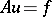(1)

is regarded as the steady-state limit solution for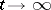of a Cauchy initial value problem for a non-stationary evolution equation involving the same operator(cf. Cauchy problem). This evolution equation may e.g. be of the form(2)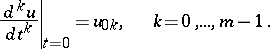Here the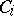are suitable operators which guarantee the existence of the "adjustment limit"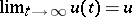.

A result of using adjustment is that it permits one to use approximate solution methods of (2) in order to construct iteration algorithms for solving equation (1) (cf. Iteration algorithm). Thus, for the non-stationary equation (2) one could employ a discretization (differencing) with respect tosolution method which is convergent and stable to obtain approximate solutions. For example, for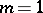, an explicit method of the form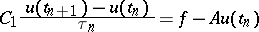where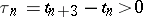. And then this method can be interpreted as an iteration algorithm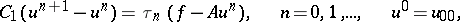for solving equation (1), in whichand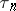are now seen as characterizing this (iteration) method.

Varying the form of the operatorsand considering different discretizations with respect toin equation (2) (explicit schemes, implicit schemes, splitting schemes, etc.) gives the possibility of obtaining a wide variety of iteration methods for solving equation (1). For these methods equation (2) will be the closure of the computational algorithm (cf. Closure of a computational algorithm). A generalization of the adjustment method is the continuation method (to a parametrized family).

How to Cite This Entry: